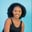Related Tags

numpy
python3

# How to use the np.modf() function for a 2D array in PythonMaria Elijah

## The numpy.modf() function

The Python library, NumPy, has a method called modf(). The numpy.modf() method returns the fractional and integral parts of an input array, element-wise.

Note: A two-dimensional (2D) array can be created using a list of lists in Python.

### Syntax

numpy.modf(x1, x2, dtype=None, out=None)


### Parameters

• x1: This is an array-like parameter that represents the input data.
• dtype: This is an optional parameter. It represents the return type of the array.
• out: This is an optional parameter. It represents the alternative output array in which the result is to be placed.

### Return value

The numpy.modf() method returns the fractional and integral parts of an input array, element-wise.

Note: If the specified number is negative, the fractional and integral parts are negative.

### Code

The following code shows how to use the numpy.modf() method for two-dimensional (2D) arrays:

# import numpy
import numpy as np
# create a 2D arrays
x = np.array([[2,-0.6,5],[3.0,4,8.23]])

# using modf()
result = np.modf(x)

print(result)

### Explanation

• Line 2: We import the numpy library.
• Line 4: We create a 2D array called x.
• Line 7: We use the np.modf() with the parameter x. This returns the fractional and integral parts of an input array element-wise. The result is stored in a new variable called result.
• Line 9: We display the result.

RELATED TAGS

numpy
python3

CONTRIBUTORMaria Elijah
RELATED COURSES

View all Courses

Keep Exploring

Learn in-demand tech skills in half the time